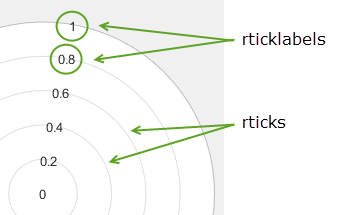# rticks

## 语法

``rticks(ticks)``
``rt = rticks``
``rticks('auto')``
``rticks('manual')``
``m = rticks('mode')``
``___ = rticks(pax,___)``

## 说明

``rticks(ticks)` 设置 r 轴刻度值，即 r 轴上显示刻度线和网格线的位置。指定 `ticks` 为递增值向量；例如 `[0 2 4 6]`。此命令作用于当前坐标区。`
``rt = rticks` 以向量的形式返回当前 r 轴刻度值。`

``rticks('auto')` 设置自动模式，从而允许坐标区确定 r 轴刻度值。如果您更改了刻度值，然后又想将它们设置回默认值，则可以使用此选项。 `
``rticks('manual')` 设置手动模式，从而将 r 轴刻度值冻结在当前值。如果您希望在调整坐标区大小或者向坐标区添加新数据时保留当前刻度值，则可以使用此选项。`
``m = rticks('mode')` 返回当前 r 轴刻度标签模式，即 `'auto'` 或 `'manual'`。默认情况下，模式为自动，除非您指定刻度值或将模式更改为手动。`

``___ = rticks(pax,___)` 使用坐标区对象 `pax`，而不是当前坐标区。指定 `pax` 作为上述任何语法的第一个输入参数。`

## 示例

```theta = linspace(0,2*pi); rho = theta/10; polarplot(theta,rho) rticks([0.1 0.25 0.5]) rticklabels({'r = .1','r = .3','r = .5'})```r 轴上 0 和 10 之间的非均匀分布的值处显示刻度线和网格线。MATLAB® 用数值标注刻度线。

```theta = 0:0.01:2*pi; rho = 10*sin(2*theta); polarplot(theta,rho) rticks([0 4 5 6 10])``````theta = 0:0.01:2*pi; rho = 50*sin(2*theta); polarplot(theta,rho) rticks(0:10:50)``````polarplot(1:10) rticks([0 5 10])````rticks('auto')````pax = polaraxes; rticks(pax,[0 .5 1])``````polarplot(1:100) rticks([])```## 输出参数

• `'auto'` - 坐标区自动确定 r 轴刻度值。

• `'manual'` - 坐标区使用手动指定的 r 轴刻度值。

## 详细信息

### 刻度值## 算法

`rticks` 函数可设置和查询与 r 轴刻度值相关的多个坐标区属性。